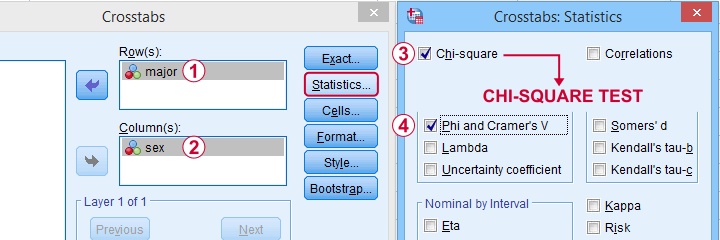SPSS TUTORIALS BASICS ANOVA REGRESSION FACTOR CORRELATION

# SPSS Chi-Square Test Tutorials## Chi-Square Independence Test

### Chi-Square Independence Test – What and Why?

A chi-square independence test evaluates if two categorical variables are related in some population.

This simple introduction explains how the test basically works and how to run and interpret it.

### SPSS Chi-Square Independence Test Tutorial

SPSS chi-square independence test examines if two categorical variables are associated. For the strength of such an association, inspect Cramér’s V, Kendall’s tau or the Spearman correlation.

### SPSS Chi-Square Test with Pairwise Z-Tests

Most data analysts are familiar with post hoc tests for ANOVA. Oddly, such post hoc tests for the chi-square independence test are not widely used.

This tutorial walks you through 2 options for obtaining and interpreting them in SPSS.

## Chi-Square Goodness-of-Fit Test

### Chi-Square Goodness-of-Fit Test – Simple Tutorial

A chi-square goodness-of-fit test examines if a categorical variable has some hypothesized frequency distribution in some population.

### SPSS One Sample Chi-Square Test

SPSS one-sample chi-square test is used to test whether a single categorical variable follows a hypothesized population distribution.

# Tell us what you think!

*Required field. Your comment will show up after approval from a moderator.

# THIS TUTORIAL HAS 2 COMMENTS:

•### By A. F. Yusuf on September 8th, 2018

Want to learn SPSS

•### By K.C.T. on November 28th, 2018

The bottom line given instantly. Hard to find elsewhere.